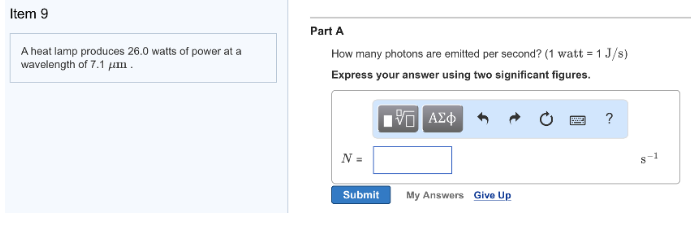# Problem: Part A A heat lamp produces 26.0 watts of power at a wavelength of 7.1 μm.How many photons are emitted per second? (1 watt = 1J/s) Express your answer using two significant figures.

###### FREE Expert Solution
80% (209 ratings)###### Problem Details

Part A

A heat lamp produces 26.0 watts of power at a wavelength of 7.1 μm.

How many photons are emitted per second? (1 watt = 1J/s)